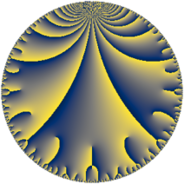# Properties

 Label 43.9.dLevel $43$ Weight $9$ Character orbit 43.d Rep. character $\chi_{43}(7,\cdot)$ Character field $\Q(\zeta_{6})$ Dimension $56$ Newform subspaces $1$ Sturm bound $33$ Trace bound $0$

# Related objects

## Defining parameters

 Level: $$N$$ $$=$$ $$43$$ Weight: $$k$$ $$=$$ $$9$$ Character orbit: $$[\chi]$$ $$=$$ 43.d (of order $$6$$ and degree $$2$$) Character conductor: $$\operatorname{cond}(\chi)$$ $$=$$ $$43$$ Character field: $$\Q(\zeta_{6})$$ Newform subspaces: $$1$$ Sturm bound: $$33$$ Trace bound: $$0$$

## Dimensions

The following table gives the dimensions of various subspaces of $$M_{9}(43, [\chi])$$.

Total New Old
Modular forms 60 60 0
Cusp forms 56 56 0
Eisenstein series 4 4 0

## Trace form

 $$56q - 84q^{3} - 6474q^{4} - 3q^{5} + 1791q^{6} - 5031q^{7} + 57858q^{9} + O(q^{10})$$ $$56q - 84q^{3} - 6474q^{4} - 3q^{5} + 1791q^{6} - 5031q^{7} + 57858q^{9} + 12491q^{10} + 31928q^{11} + 56574q^{12} - 24868q^{13} + 106830q^{14} - 11989q^{15} + 655366q^{16} + 5963q^{17} + 326445q^{18} - 67476q^{19} - 713343q^{20} - 187232q^{21} - 210724q^{23} - 1651574q^{24} + 1650473q^{25} + 765q^{26} + 684492q^{28} - 5568699q^{29} - 3412593q^{30} + 2602959q^{31} - 554514q^{33} - 584967q^{34} + 8137398q^{35} - 3035428q^{36} - 3541812q^{37} + 6280356q^{38} - 2473841q^{40} + 7375370q^{41} + 15490227q^{43} - 2983894q^{44} + 3217584q^{46} - 7588210q^{47} + 5844987q^{48} + 33155723q^{49} - 9064422q^{50} + 3837506q^{52} - 5366122q^{53} - 45158560q^{54} + 40061478q^{55} - 39705477q^{56} + 13330347q^{57} + 19271684q^{58} + 18367244q^{59} - 36808121q^{60} - 6722484q^{61} - 15483591q^{62} - 40574925q^{63} - 87236036q^{64} - 33263013q^{66} - 8581935q^{67} - 123045082q^{68} - 26516709q^{69} - 132304098q^{71} - 100762446q^{72} + 278404467q^{73} + 182026410q^{74} + 179327130q^{76} + 143930505q^{77} - 143413472q^{78} - 62931368q^{79} + 121842717q^{80} - 65032540q^{81} - 29005780q^{83} + 370916864q^{84} + 73281798q^{86} + 137580504q^{87} - 124655943q^{89} + 63835252q^{90} + 287393496q^{91} - 215232271q^{92} - 124327923q^{93} + 413979855q^{95} + 51391714q^{96} - 446400270q^{97} - 351696195q^{98} - 146664065q^{99} + O(q^{100})$$

## Decomposition of $$S_{9}^{\mathrm{new}}(43, [\chi])$$ into newform subspaces

Label Dim. $$A$$ Field CM Traces $q$-expansion
$$a_2$$ $$a_3$$ $$a_5$$ $$a_7$$
43.9.d.a $$56$$ $$17.517$$ None $$0$$ $$-84$$ $$-3$$ $$-5031$$